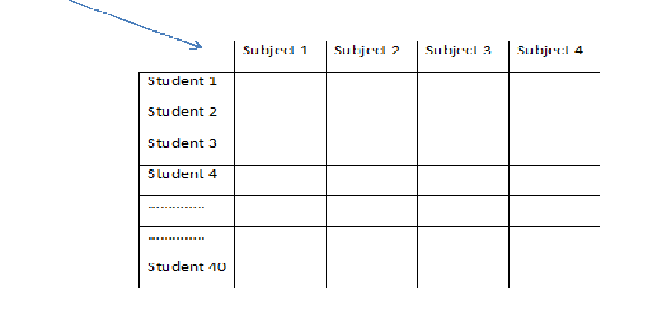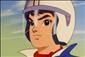# Arrays in Java: Multidimensional Arrays (Part-4)

Multidimensional arrays, as the name suggests, contain more than one dimension. You can create two-dimensional, three-dimensional, and n-dimensional arrays in Java (where n is any natural number). The number of dimensions may be any large number, subject to the restrictions imposed by the compiler. The JDK compiler puts this limit at 255, which is so large that developers need not worry about it.

##### Two-dimensional Arrays

A two-dimensional array can be visualized as a table consisting of rows and columns. Each cell of the table denotes an array element.

The general syntax for declaring a two-dimensional array is as follows:

type arrayName [ ] [ ] ;

or

type [ ] [ ] arrayName ;

The type specifies the type of data that each array element will hold. The arrayName specifies the name for the array. The two square brackets indicate that the current array variable declaration refers to a two-dimensional array.

Suppose we have to write a program to store marks obtained by each student in a class in various subjects. Let’s assume that each student takes four subjects and that 40 students are in the class. We will need to create a two-dimensional array to store this data. We would write the following block of code to create such an rray:

```final int NUMBER_OF_SUBJECTS = 5;
final int NUMBER_OF_STUDENTS = 40;
int[ ][ ] studentMarks;
studentMarks = new int[NUMBER_OF_SUBJECTS][NUMBER_OF_STUDENTS];```

The first dimension of the array (that is, the row) specifies the subject ID, and the second dimension (that is, the column) specifies the student ID. We could easily interchange rows and columns in the preceding declaration by changing their order of declaration as shown in the following statement:

studentMarks = new int[NUMBER_OF_STUDENTS][NUMBER_OF_SUBJECTS];

In this case, the first dimension specifies the student ID and the second dimension specifies the subject ID.The tabular map displayed here shows both student and subject’s starting with 1. (Generally, a student and a subject will not be assigned an ID equal to zero.) When we store the student marks in this array, we will need to adjust both indices by 1 to comply with Java’s requirements of array indexing. For example, to assign the marks obtained by student 7 in subject 3, you will use the following syntax:

marks = 88;

Note that a row-index value of 6 indicates the seventh row in the table because the index always starts with zero. Similarly, a column-index value of 2 indicates the third column in the table.

As another example, the syntax marks would denote the marks obtained by a student 1 in subject 1. (Again, remember that the student IDs and subject numbers start with 1 in our notation.) Similarly, marks would denote the marks obtained by the last student in the class (student ID 50) in the last subject (subject ID 4).

##### Initializing at Runtime

To initialize an element of a two-dimensional array, we use the syntax discussed earlier for accessing the element and then use an assignment statement to initialize it:

arrayName [ row ] [ col ] = data ;

For example, consider the following declaration for a two-dimensional array of integers:

int[ ][ ] marks;

marks = new int;

The individual elements of this array may be initialized using following program statements:

marks = 69;

marks = 86;

##### Initializing Using Array Literals

We may define the values of individual array elements in curly braces on the right side of the assignment operator during the array declaration. The general syntax for initializing elements of a two-dimensional array using this method is shown here:

int[ ][ ] subjectMarks = { {1, 98}, {2, 58}, {3, 78},{4, 89}    ………};

Note the use of nested curly braces to separate out the different rows of the data declarations. The Java compiler will generate the bytecode for the preceding declaration that is the equivalent of the following:

```subjectMarks = new int[ ][ ];
subjectMarks = 1;
subjectMarks = 98;
subjectMarks = 2;```
Last updated:9/7/2019 12:16:19 AM#### David Miller